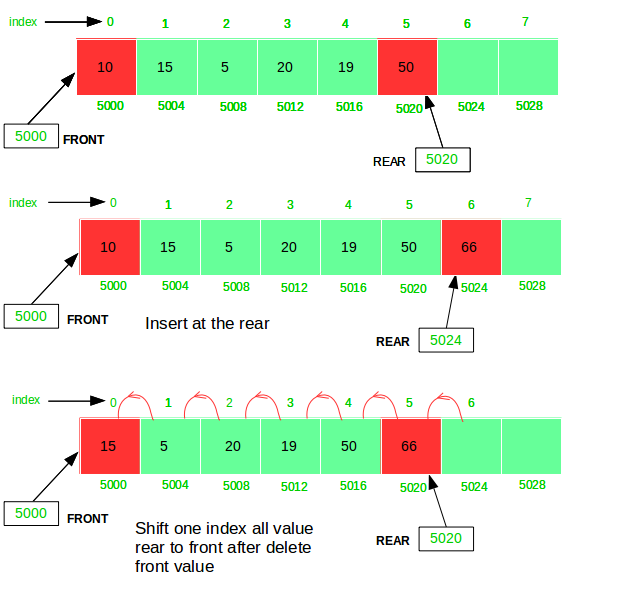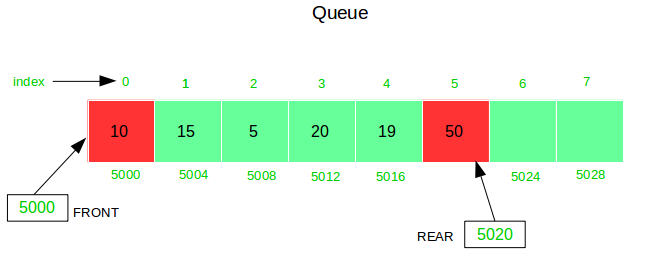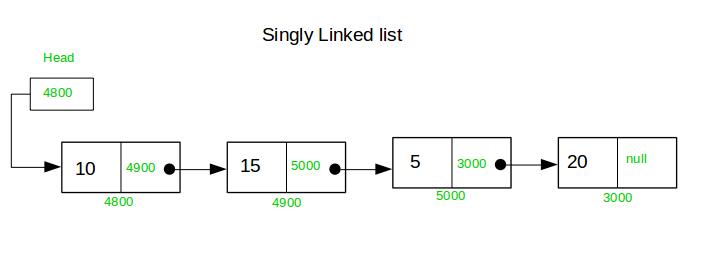# Difference between a Static Queue and a Singly Linked List

Static Queue: A queue is an ordered list of elements. It always works in first in first out(FIFO) fashion. All the elements get inserted at the REAR and removed from the FRONT of the queue. In implementation of the static Queue, an array will be used so all operation of queue are index based which makes it faster for all operations except deletion because deletion requires shifting of all the remaining elements to the front by one position.

A Static Queue is a queue of fixed size implemented using array.Singly Linked List: A linked list is also an ordered list of elements. You can add an element anywhere in the list, change an element anywhere in the list, or remove an element from any position in the list. Each node in the list stores the content and a pointer or reference to the next node in the list. To store a single linked list, only the reference or pointer to the first node in that list must be stored. The last node in a single linked list points to nothing (or null).Here are some of the major differences between a Static Queue and a Singly Linked List

Queue is a collection of one or more elements arranged in memory in a contiguous fashion. A linked list is a collection of one or more elements arranged in memory in a dis-contiguous fashion.
Static Queue is always fixed size. List size is never fixed.
In Queue, only one and single type of information is stored because static Queue implementation is through Array. List also stored the address for the next node along with it’s content.
Static Queue is index based. Singly linked list is reference based.
Insertion can always be performed on a single end called REAR and deletion on the other end called FRONT. Insertion as well as deletion can performed any where within the list.
Queue is always based on FIFO. List may be based on FIFI or LIFO etc.
Queue have two pointer FRONT and REAR. While List has only one pointer basically called HEAD.

Below is the implementation of a Static Queue:

## Java

 `// Java program to implement a queue using an array ` `class` `Queue { ` `    ``private` `static` `int` `front, rear, capacity; ` `    ``private` `static` `int` `queue[]; ` ` `  `    ``Queue(``int` `c) ` `    ``{ ` `        ``front = rear = ``0``; ` `        ``capacity = c; ` `        ``queue = ``new` `int``[capacity]; ` `    ``} ` ` `  `    ``// function to insert an element ` `    ``// at the rear of the queue ` `    ``static` `void` `queueEnqueue(``int` `data) ` `    ``{ ` `        ``// check queue is full or not ` `        ``if` `(capacity == rear) { ` `            ``System.out.printf(``"\nQueue is full\n"``); ` `            ``return``; ` `        ``} ` ` `  `        ``// insert element at the rear ` `        ``else` `{ ` `            ``queue[rear] = data; ` `            ``rear++; ` `        ``} ` `        ``return``; ` `    ``} ` ` `  `    ``// function to delete an element ` `    ``// from the front of the queue ` `    ``static` `void` `queueDequeue() ` `    ``{ ` `        ``// if queue is empty ` `        ``if` `(front == rear) { ` `            ``System.out.printf(``"\nQueue is empty\n"``); ` `            ``return``; ` `        ``} ` ` `  `        ``// shift all the elements from index 2 till rear ` `        ``// to the right by one ` `        ``else` `{ ` `            ``for` `(``int` `i = ``0``; i < rear - ``1``; i++) { ` `                ``queue[i] = queue[i + ``1``]; ` `            ``} ` ` `  `            ``// store 0 at rear indicating there's no element ` `            ``if` `(rear < capacity) ` `                ``queue[rear] = ``0``; ` ` `  `            ``// decrement rear ` `            ``rear--; ` `        ``} ` `        ``return``; ` `    ``} ` ` `  `    ``// print queue elements ` `    ``static` `void` `queueDisplay() ` `    ``{ ` `        ``int` `i; ` `        ``if` `(front == rear) { ` `            ``System.out.printf(``"\nQueue is Empty\n"``); ` `            ``return``; ` `        ``} ` ` `  `        ``// traverse front to rear and print elements ` `        ``for` `(i = front; i < rear; i++) { ` `            ``System.out.printf(``" %d <-- "``, queue[i]); ` `        ``} ` `        ``return``; ` `    ``} ` ` `  `    ``// print front of queue ` `    ``static` `void` `queueFront() ` `    ``{ ` `        ``if` `(front == rear) { ` `            ``System.out.printf(``"\nQueue is Empty\n"``); ` `            ``return``; ` `        ``} ` `        ``System.out.printf(``"\nFront Element is: %d"``, queue[front]); ` `        ``return``; ` `    ``} ` `} ` ` `  `public` `class` `StaticQueueinjava { ` ` `  `    ``// Driver code ` `    ``public` `static` `void` `main(String[] args) ` `    ``{ ` `        ``// Create a queue of capacity 4 ` `        ``Queue q = ``new` `Queue(``4``); ` ` `  `        ``// print Queue elements ` `        ``q.queueDisplay(); ` ` `  `        ``// inserting elements in the queue ` `        ``q.queueEnqueue(``20``); ` `        ``q.queueEnqueue(``30``); ` `        ``q.queueEnqueue(``40``); ` `        ``q.queueEnqueue(``50``); ` ` `  `        ``// print Queue elements ` `        ``q.queueDisplay(); ` ` `  `        ``// insert element in the queue ` `        ``q.queueEnqueue(``60``); ` ` `  `        ``// print Queue elements ` `        ``q.queueDisplay(); ` ` `  `        ``q.queueDequeue(); ` `        ``q.queueDequeue(); ` `        ``System.out.printf(``"\n\nafter two node deletion\n\n"``); ` ` `  `        ``// print Queue elements ` `        ``q.queueDisplay(); ` ` `  `        ``// print front of the queue ` `        ``q.queueFront(); ` `    ``} ` `} `

## C#

 `// C# program to implement a queue using an array ` `using` `System;  ` `     `  `public` `class` `Queue  ` `{ ` `    ``private` `static` `int` `front, rear, capacity; ` `    ``private` `static` `int` `[]queue; ` ` `  `    ``public` `Queue(``int` `c) ` `    ``{ ` `        ``front = rear = 0; ` `        ``capacity = c; ` `        ``queue = ``new` `int``[capacity]; ` `    ``} ` ` `  `    ``// function to insert an element ` `    ``// at the rear of the queue ` `    ``public` `void` `queueEnqueue(``int` `data) ` `    ``{ ` `        ``// check queue is full or not ` `        ``if` `(capacity == rear)  ` `        ``{ ` `            ``Console.Write(``"\nQueue is full\n"``); ` `            ``return``; ` `        ``} ` ` `  `        ``// insert element at the rear ` `        ``else` `        ``{ ` `            ``queue[rear] = data; ` `            ``rear++; ` `        ``} ` `        ``return``; ` `    ``} ` ` `  `    ``// function to delete an element ` `    ``// from the front of the queue ` `    ``public` `void` `queueDequeue() ` `    ``{ ` `        ``// if queue is empty ` `        ``if` `(front == rear)  ` `        ``{ ` `            ``Console.Write(``"\nQueue is empty\n"``); ` `            ``return``; ` `        ``} ` ` `  `        ``// shift all the elements from index 2 till rear ` `        ``// to the right by one ` `        ``else` `        ``{ ` `            ``for` `(``int` `i = 0; i < rear - 1; i++)  ` `            ``{ ` `                ``queue[i] = queue[i + 1]; ` `            ``} ` ` `  `            ``// store 0 at rear indicating there's no element ` `            ``if` `(rear < capacity) ` `                ``queue[rear] = 0; ` ` `  `            ``// decrement rear ` `            ``rear--; ` `        ``} ` `        ``return``; ` `    ``} ` ` `  `    ``// print queue elements ` `    ``public` `void` `queueDisplay() ` `    ``{ ` `        ``int` `i; ` `        ``if` `(front == rear)  ` `        ``{ ` `            ``Console.Write(``"\nQueue is Empty\n"``); ` `            ``return``; ` `        ``} ` ` `  `        ``// traverse front to rear and print elements ` `        ``for` `(i = front; i < rear; i++) ` `        ``{ ` `            ``Console.Write(``" {0} <-- "``, queue[i]); ` `        ``} ` `        ``return``; ` `    ``} ` ` `  `    ``// print front of queue ` `    ``public` `void` `queueFront() ` `    ``{ ` `        ``if` `(front == rear)  ` `        ``{ ` `            ``Console.Write(``"\nQueue is Empty\n"``); ` `            ``return``; ` `        ``} ` `        ``Console.Write(``"\nFront Element is: {0}"``, queue[front]); ` `        ``return``; ` `    ``} ` `} ` ` `  `public` `class` `StaticQueueinjava  ` `{ ` ` `  `    ``// Driver code ` `    ``public` `static` `void` `Main(String[] args) ` `    ``{ ` `        ``// Create a queue of capacity 4 ` `        ``Queue q = ``new` `Queue(4); ` ` `  `        ``// print Queue elements ` `        ``q.queueDisplay(); ` ` `  `        ``// inserting elements in the queue ` `        ``q.queueEnqueue(20); ` `        ``q.queueEnqueue(30); ` `        ``q.queueEnqueue(40); ` `        ``q.queueEnqueue(50); ` ` `  `        ``// print Queue elements ` `        ``q.queueDisplay(); ` ` `  `        ``// insert element in the queue ` `        ``q.queueEnqueue(60); ` ` `  `        ``// print Queue elements ` `        ``q.queueDisplay(); ` ` `  `        ``q.queueDequeue(); ` `        ``q.queueDequeue(); ` `        ``Console.Write(``"\n\nafter two node deletion\n\n"``); ` ` `  `        ``// print Queue elements ` `        ``q.queueDisplay(); ` ` `  `        ``// print front of the queue ` `        ``q.queueFront(); ` `    ``} ` `} ` ` `  `/* This code contributed by PrinciRaj1992 */`

Output:

```Queue is Empty
20 <--  30 <--  40 <--  50 <--
Queue is full
20 <--  30 <--  40 <--  50 <--

after two node deletion

40 <--  50 <--
Front Element is: 40
```

Below is the implementation of a Singly Linked List:

## Java

 `// Java program to implement singly linked list ` `class` `SinglyLList { ` ` `  `    ``class` `Node { ` ` `  `        ``// node variables ` `        ``int` `data; ` `        ``Node next; ` ` `  `        ``public` `Node(``int` `data) ` `        ``{ ` `            ``this``.data = data; ` `            ``this``.next = ``null``; ` `        ``} ` `    ``} ` ` `  `    ``// create reference variable of Node ` `    ``Node head; ` ` `  `    ``// function to insert a node ` `    ``// at the beginning of the list ` `    ``void` `InsertAtStart(``int` `data) ` `    ``{ ` `        ``// create a node ` `        ``Node new_node = ``new` `Node(data); ` ` `  `        ``new_node.next = head; ` `        ``head = new_node; ` `    ``} ` ` `  `    ``// function to insert node ` `    ``// at the end of the list ` `    ``void` `InsertAtLast(``int` `data) ` `    ``{ ` ` `  `        ``Node new_node = ``new` `Node(data); ` `        ``if` `(head == ``null``) { ` `            ``head = new_node; ` `            ``return``; ` `        ``} ` ` `  `        ``new_node.next = ``null``; ` ` `  `        ``Node last = head; ` `        ``while` `(last.next != ``null``) { ` `            ``last = last.next; ` `        ``} ` ` `  `        ``last.next = new_node; ` `    ``} ` ` `  `    ``// function to delete a node ` `    ``// at the beginning of the list ` `    ``void` `DeleteAtStart() ` `    ``{ ` `        ``if` `(head == ``null``) { ` `            ``System.out.println(``"List is empty"``); ` `            ``return``; ` `        ``} ` `        ``head = head.next; ` `    ``} ` ` `  `    ``// function to delete a node at ` `    ``// a given position in the list ` `    ``void` `DeleteAtPos(``int` `pos) ``throws` `Exception ` `    ``{ ` `        ``int` `position = ``0``; ` `        ``if` `(pos > Count() || pos < ``0``) { ` `            ``throw` `new` `Exception(``"Incorrect position exception"``); ` `        ``} ` `        ``Node temp = head; ` `        ``while` `(position != pos - ``1``) { ` `            ``temp = temp.next; ` `            ``position++; ` `        ``} ` `        ``temp.next = temp.next.next; ` `    ``} ` ` `  `    ``// function to delete a node ` `    ``// from the end of the list ` `    ``void` `DeleteAtLast() ` `    ``{ ` `        ``Node delete = head; ` `        ``while` `(delete.next != ``null` `               ``&& delete.next.next != ``null``) { ` `            ``delete = delete.next; ` `        ``} ` `        ``delete.next = ``null``; ` `    ``} ` ` `  `    ``// function to display all the nodes of the list ` `    ``void` `Display() ` `    ``{ ` `        ``Node disp = head; ` `        ``while` `(disp != ``null``) { ` `            ``System.out.print(disp.data + ``"->"``); ` `            ``disp = disp.next; ` `        ``} ` `    ``} ` ` `  `    ``// function to return the total nodes in the list ` `    ``int` `Count() ` `    ``{ ` `        ``int` `elements = ``0``; ` `        ``Node count = head; ` `        ``while` `(count != ``null``) { ` `            ``count = count.next; ` `            ``elements++; ` `        ``} ` `        ``return` `elements; ` `    ``} ` `} ` ` `  `public` `class` `GFG { ` ` `  `    ``// Driver code ` `    ``public` `static` `void` `main(String[] args) ``throws` `Exception ` `    ``{ ` `        ``// create object of class singlyList ` `        ``SinglyLList list = ``new` `SinglyLList(); ` ` `  `        ``// insert elements of singly linked list ` `        ``// at beginning ` `        ``list.InsertAtStart(``3``); ` `        ``list.InsertAtStart(``2``); ` `        ``list.InsertAtStart(``1``); ` ` `  `        ``// print linked list elements ` `        ``list.Display(); ` ` `  `        ``// insert element at the end of list ` ` `  `        ``list.InsertAtLast(``1``); ` `        ``System.out.println(``"\nafter inserting node at the end\n "``); ` ` `  `        ``// print linked list elements ` `        ``list.Display(); ` ` `  `        ``// delete an element at the given position ` `        ``list.DeleteAtPos(``1``); ` ` `  `        ``// delete starting element ` `        ``list.DeleteAtStart(); ` ` `  `        ``// delete last element ` `        ``list.DeleteAtLast(); ` ` `  `        ``System.out.println(``"\nafter deleting node: second, first and last\n "``); ` ` `  `        ``// print linked list elements ` `        ``list.Display(); ` `    ``} ` `} `

## C#

 `// C# program to implement singly linked list  ` `using` `System; ` ` `  `public` `class` `SinglyLList  ` `{  ` ` `  `    ``public` `class` `Node ` `    ``{  ` ` `  `        ``// node variables  ` `        ``public` `int` `data;  ` `        ``public` `Node next;  ` ` `  `        ``public` `Node(``int` `data)  ` `        ``{  ` `            ``this``.data = data;  ` `            ``this``.next = ``null``;  ` `        ``}  ` `    ``}  ` ` `  `    ``// create reference variable of Node  ` `    ``public` `Node head;  ` ` `  `    ``// function to insert a node  ` `    ``// at the beginning of the list  ` `    ``public` `void` `InsertAtStart(``int` `data)  ` `    ``{  ` `        ``// create a node  ` `        ``Node new_node = ``new` `Node(data);  ` ` `  `        ``new_node.next = head;  ` `        ``head = new_node;  ` `    ``}  ` ` `  `    ``// function to insert node  ` `    ``// at the end of the list  ` `    ``public` `void` `InsertAtLast(``int` `data)  ` `    ``{  ` ` `  `        ``Node new_node = ``new` `Node(data);  ` `        ``if` `(head == ``null``)  ` `        ``{  ` `            ``head = new_node;  ` `            ``return``;  ` `        ``}  ` ` `  `        ``new_node.next = ``null``;  ` ` `  `        ``Node last = head;  ` `        ``while` `(last.next != ``null``) ` `        ``{  ` `            ``last = last.next;  ` `        ``}  ` ` `  `        ``last.next = new_node;  ` `    ``}  ` ` `  `    ``// function to delete a node  ` `    ``// at the beginning of the list  ` `    ``public` `void` `DeleteAtStart()  ` `    ``{  ` `        ``if` `(head == ``null``)  ` `        ``{  ` `            ``Console.WriteLine(``"List is empty"``);  ` `            ``return``;  ` `        ``}  ` `        ``head = head.next;  ` `    ``}  ` ` `  `    ``// function to delete a node at  ` `    ``// a given position in the list  ` `    ``public` `void` `DeleteAtPos(``int` `pos)  ` `    ``{  ` `        ``int` `position = 0;  ` `        ``if` `(pos > Count() || pos < 0) ` `        ``{  ` `            ``throw` `new` `Exception(``"Incorrect position exception"``);  ` `        ``}  ` `        ``Node temp = head;  ` `        ``while` `(position != pos - 1)  ` `        ``{  ` `            ``temp = temp.next;  ` `            ``position++;  ` `        ``}  ` `        ``temp.next = temp.next.next;  ` `    ``}  ` ` `  `    ``// function to delete a node  ` `    ``// from the end of the list  ` `    ``public` `void` `DeleteAtLast()  ` `    ``{  ` `        ``Node delete = head;  ` `        ``while` `(delete.next != ``null` `            ``&& delete.next.next != ``null``)  ` `        ``{  ` `            ``delete = delete.next;  ` `        ``}  ` `        ``delete.next = ``null``;  ` `    ``}  ` ` `  `    ``// function to display all the nodes of the list  ` `    ``public` `void` `Display()  ` `    ``{  ` `        ``Node disp = head;  ` `        ``while` `(disp != ``null``) ` `        ``{  ` `            ``Console.Write(disp.data + ``"->"``);  ` `            ``disp = disp.next;  ` `        ``}  ` `    ``}  ` ` `  `    ``// function to return the total nodes in the list  ` `    ``public` `int` `Count()  ` `    ``{  ` `        ``int` `elements = 0;  ` `        ``Node count = head;  ` `        ``while` `(count != ``null``) ` `        ``{  ` `            ``count = count.next;  ` `            ``elements++;  ` `        ``}  ` `        ``return` `elements;  ` `    ``}  ` `}  ` ` `  `class` `GFG  ` `{  ` ` `  `    ``// Driver code  ` `    ``public` `static` `void` `Main(String[] args) ` `    ``{  ` `        ``// create object of class singlyList  ` `        ``SinglyLList list = ``new` `SinglyLList();  ` ` `  `        ``// insert elements of singly linked list  ` `        ``// at beginning  ` `        ``list.InsertAtStart(3);  ` `        ``list.InsertAtStart(2);  ` `        ``list.InsertAtStart(1);  ` ` `  `        ``// print linked list elements  ` `        ``list.Display();  ` ` `  `        ``// insert element at the end of list  ` ` `  `        ``list.InsertAtLast(1);  ` `        ``Console.WriteLine(``"\nafter inserting node at the end\n "``);  ` ` `  `        ``// print linked list elements  ` `        ``list.Display();  ` ` `  `        ``// delete an element at the given position  ` `        ``list.DeleteAtPos(1);  ` ` `  `        ``// delete starting element  ` `        ``list.DeleteAtStart();  ` ` `  `        ``// delete last element  ` `        ``list.DeleteAtLast();  ` ` `  `        ``Console.WriteLine(``"\nafter deleting node: second, first and last\n "``);  ` ` `  `        ``// print linked list elements  ` `        ``list.Display();  ` `    ``}  ` `}  ` ` `  `// This code has been contributed by 29AjayKumar `

Output:

```1->2->3->
after inserting node at the end

1->2->3->1->
after deleting node: second, first and last

3->
```

My Personal Notes arrow_drop_upCheck out this Author's contributed articles.

If you like GeeksforGeeks and would like to contribute, you can also write an article using contribute.geeksforgeeks.org or mail your article to contribute@geeksforgeeks.org. See your article appearing on the GeeksforGeeks main page and help other Geeks.

Please Improve this article if you find anything incorrect by clicking on the "Improve Article" button below.